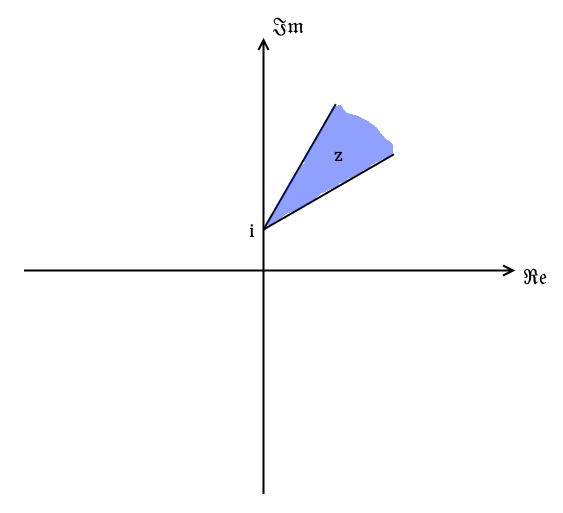# Further complex numbers

## Introduction

Recall from FP1 that a complex number $z$ can be expressed in modulus-argument form

$$z = r\left(\cos\theta +i\sin\theta\right)$$

where $r=|z|$ and $\theta = \arg(z)$.

In FP2 we extend this notion by expressing complex numbers in exponential form, and using the $\sin$ and $\cos$ relations to come up with new trig formulae.

## Exponential form

Let $z$ be a complex number. Then $z$ can be expressed in the form

$$z = r e^{i\theta}$$

where $r=|z|$ and $\theta = \arg(z)$.

The Maclaurin series for $\cos\theta$, $\sin\theta$, and $e^{x}$ are

\begin{align} \cos\theta &= 1 - \frac{\theta^{2}}{2!} + \frac{\theta^{4}}{4!} - \frac{\theta^{6}}{6!} + \ldots \\ \sin\theta &= \theta - \frac{\theta^{3}}{3!} + \frac{\theta^{5}}{5!} - \frac{\theta^{7}}{7!} + \ldots \\ e^{x} &= 1+ x+\frac{x^{2}}{2!}+\frac{x^{3}}{3!}+\ldots \end{align}

Let $x=i\theta$. Then

\begin{align} e^{i\theta} &= 1+ i\theta+\frac{\left(i\theta\right)^{2}}{2!}+\frac{\left(i\theta\right)^{3}}{3!}+\frac{\left(i\theta\right)^{4}}{4!}+\frac{\left(i\theta\right)^{5}}{5!}+\ldots \\ &= 1 + i\theta-\frac{\theta^{2}}{2!}-\frac{i\theta^{3}}{3!}+\frac{\theta^{4}}{4!}+\frac{i\theta^{5}}{5!}+\ldots \\ &= 1 -\frac{\theta^{2}}{2!} +\frac{\theta^{4}}{4!} + \ldots + i\left[\theta -\frac{\theta^{3}}{3!}+\frac{\theta^{5}}{5!}+\ldots \right] \\ &= \cos\theta + i\sin\theta ~ \blacksquare \end{align}

This relationship $e^{i\theta} = \cos\theta + i\sin\theta$ is called Euler's formula.

As with the modulus-argument form, the exponential form makes complex number multiplication and division extremely convenient. Take two complex numbers $z_1$ and $z_2$. Then

$$z_1 \cdot z_2 = r_1 e^{i\theta_1} \cdot r_2 e^{i\theta_2} = r_1 r_2 e ^{i\left(\theta_1 + \theta_2\right)}$$

And

$$\frac{z_1}{z_2} = \frac{r_1 e^{i\theta_1}}{r_2 e^{i\theta_2}} = \frac{r_1}{r_2} e ^{i\left(\theta_1 - \theta_2\right)}$$

So the operations just follow the normal behaviour of the $e^x$ function.

### Example

Q) Find $\frac{3+4i}{5-12i}$.

A) Firstly $|3+4i| = 5$ and $\arg(3+4i) = 0.927^c$ (3.s.f.). Then $|5-12i| = 13$ and $\arg(5-12i) = -1.18^c$ (3.s.f.). So we have

$$\frac{3+4i}{5-12i} = \frac{5e^{0.927i}}{13e^{-1.18i}} = \frac{5}{13}e^{2.11i}$$

## De Moivre's theorem

De Moivre's theorem states that $$\left(\cos x + i\sin x \right)^{n} = \cos(nx) + i\sin(nx)$$

We proved earlier that

$$e^{ix} = \cos x + i\sin x$$

So

$$\left(\cos x + i\sin x \right)^{n} = \left(e^{ix}\right)^{n} = e^{inx} = \cos(nx) + i\sin(nx) ~ \blacksquare$$

### Example

Q) Simplify $\frac{\left(\cos\frac{\pi}{5} + i\sin\frac{\pi}{5}\right)^{3}}{\left(\cos\frac{2\pi}{3} + i\sin\frac{2\pi}{3}\right)^{2}}$.

A) By de Moivre's theorem

$$\frac{\left(\cos\frac{\pi}{5} + i\sin\frac{\pi}{5}\right)^{3}}{\left(\cos\frac{2\pi}{3} + i\sin\frac{2\pi}{3}\right)^{2}} = \frac{e^{i\frac{3\pi}{5}}}{e^{i\frac{4\pi}{3}}} = e^{-i\frac{11\pi}{15}}$$

We can also express this in terms of $\cos$ and $\sin$

\begin{align} e^{-i\frac{11}{15}} &= \cos\left(-\frac{11\pi}{15}\right) + i\sin\left(-\frac{11\pi}{15}\right) \\ &= \cos\left(\frac{11\pi}{15}\right) - i\sin\left(\frac{11\pi}{15}\right) \end{align}

Since $\cos$ is an even function and $\sin$ is an odd function. The important part is making sure the subjects of $\cos$ and $\sin$ are the same.

### Example

Q) Express $(1+i)^{5}$ in the form $a + bi$ where $a$ and $b$ are real numbers.

A) Firstly the modulus of $1+i$ is $\sqrt{2}$ and the argument of $1+i$ is $\tan^{-1}1 = \frac{\pi}{4}$. Therefore $1+i = \sqrt{2}e^{i\frac{\pi}{4}}$.

By de Moivre's theorem,

$$(1+i)^{5} = \left(\sqrt{2}e^{i\frac{\pi}{4}}\right)^{5} = \sqrt{32}e^{i\frac{5\pi}{4}}$$

And so $a^{2}+b^{2} = 32$. Also $\pi \lt \frac{5\pi}{4} \lt \frac{3\pi}{2}$ so this new complex number is in the third quadrant. That means both $a$ and $b$ are negative.

$$\frac{5\pi}{4} = \pi + \tan^{-1}\left(\frac{b}{a}\right)$$

$$\Rightarrow \frac{b}{a} = \tan\left(\frac{\pi}{4}\right)$$

$$\Rightarrow a = b$$

The complex number with $a$ and $b$ that satisfy all of these conditions is $-4-4i$ since $(-4)^{2}+(-4)^{2}=32$.

### Example

Q) Express $\cos(3\theta)$ in terms of $\cos\theta$ and $\sin(3\theta)$ in terms of $\sin\theta$.

A) By de Moivre's theorem, and letting $c=\cos\theta$ and $s=\sin\theta$ for brevity.

\begin{align} \cos(3\theta) + i\sin(3\theta) &= \left(\cos\theta +i\sin\theta\right)^{3} \\ &= c^{3} + 3c^{2}\left(is\right)+3c\left(is\right)^{2} + \left(is\right)^{3} \\ &= c^{3} + 3ic^{2}s - 3cs^{2} - is^{3} \\ &= c^{3} -3cs^{2} + i\left[3c^{2}s-s^{3}\right] \\ &= c^{3} -3c\left(1-c^{2}\right) + i\left[3\left(1-s^{2}\right)s-s^{3}\right] \\ &= 4c^{3}-3c + i\left[3s-4s^{3} \right] \\ &= 4\cos^{3}\theta - 3\cos\theta + i\left[3\sin\theta-4\sin^{3}\theta \right] \end{align}

Comparing real and imaginary parts

\begin{align} \cos(3\theta) &= 4\cos^{3}\theta - 3\cos\theta \\ \sin(3\theta) &= 3\sin\theta-4\sin^{3}\theta \end{align}

From the formula $e^{in\theta} = \cos(n\theta) + i\sin(n\theta)$ we can derive the following two formulae for $\sin$ and $\cos$.

\begin{align} \sin(n\theta) &= \frac{e^{in\theta} - e^{-in\theta}}{2i} \\ \cos(n\theta) &= \frac{e^{in\theta} + e^{-in\theta}}{2} \end{align}

Suppose the formulae are true. Then

\begin{align} \cos(n\theta) + i\sin(n\theta) &= \frac{e^{in\theta} + e^{-in\theta}}{2} + \frac{e^{in\theta} - e^{-in\theta}}{2} \\ &= \frac{e^{in\theta} + e^{-in\theta} + e^{in\theta} - e^{-in\theta}}{2} \\ &= \frac{2e^{in\theta}}{2} \\ &= e^{in\theta} ~ \blacksquare \end{align}

### Example

Q) Show that $\sin^{4}x = \frac{1}{8}\cos(4x) -\frac{1}{4}\cos(2x) + \frac{3}{8}$.

A) From the formula we get

\begin{align} \left(2i\sin x \right)^{4} &= \left(e^{ix}-e^{-ix}\right)^{4} \\ &= \left(e^{ix}\right)^{4}+4\left(e^{ix}\right)^{3}\left(-e^{-ix}\right)+6\left(e^{ix}\right)^{2}\left(-e^{-ix}\right)^{2} + 4\left(e^{ix}\right)\left(-e^{-ix}\right)^{3} +\left(-e^{-ix}\right)^{4} \\ &= e^{4ix} - 4e^{3ix-ix} +6e^{2ix-2ix} -4e^{ix-3ix}+e^{-4ix} \\ &= e^{4ix} -4e^{2ix} +6 -4e^{-2ix}+e^{-4ix} \\ &= e^{4ix}+e^{-4ix} -4\left(e^{2ix}+e^{-2ix}\right) +6 \\ &= 2\cos(4x) -4\cos(2x) + 6 \end{align}

Therefore, since $\left(2i\right)^{4}=16$

$$\sin^{4}x = \frac{1}{8}\cos(4x) -\frac{1}{4}\cos(2x) + \frac{3}{8}$$

## $n^{th}$ roots of complex numbers

The exponential form of a complex number allows you to easily find the exponents of a complex number and also its roots, owing to the behaviour of the exponential function.

Every complex number has exactly $n$ distinct $n^{th}$ roots. Note that $f(z) = z^{1/n}$ is a "multi-valued" function - you always get $n$ roots.

(Proof to come later.)

One pretty amazing feature of these $n$ roots is that a complex number's $n^{th}$ roots always line up in a perfect circle on the Argand diagram. You will see this materialise in a second.

If we are to find the $n^{th}$ root of some complex number, then let

$$z^{n} = r e^{(i\theta+2k\pi)}$$

Where $r = |z^{n}|$, $\theta=\arg\left(z^{n}\right)$, and $k=0, 1, 2, \ldots$

The added "$+2k\pi$" comes from the properties of a complex number's argument. For any complex number, going round an entire circle (i.e. an integer multiple of $2\pi$ radians) from its argument on the Argand diagram you arrive back where you started.

Then

$$z = \sqrt[n]{r e^{(i\theta+2k\pi)}} = \sqrt[n]{r}e^{\left(i\frac{\theta+2k\pi}{n}\right)}$$

And from de Moivre's theorem

$$z = \sqrt[n]{r}e^{\left(i\frac{\theta+2k\pi}{n}\right)} = \sqrt[n]{r}\left(\cos\left(\frac{\theta+2k\pi}{n}\right)+i\sin\left(\frac{\theta+2k\pi}{n}\right) \right)$$

Then to find all $n$ of the roots we sub in integer values of $k=0, 1, 2, \ldots$ and stop when we have all $n$ distinct roots.

### Example

Q) Find the cube roots of $6+8i$.

A) Let $z^3=6+8i$. Then $|z^3|=10$ and $\arg(z^3)=0.927^c$ (3.s.f.). Then using Euler's formula

$$z^3 = 10e^{i(0.927 + 2k\pi)}$$

Then taking the cube root of both sides

\begin{align} z &= \sqrt{10e^{i(0.927 + 2k\pi)}} \\ &= \sqrt{10}e^{i\frac{0.927 + 2k\pi}{3}} \end{align}

$k=0$:

$$z = \sqrt{10}e^{i\frac{0.927}{3}} = \sqrt{10}e^{0.309i}$$

$k=1$:

$$z = \sqrt{10}e^{i\frac{0.927+2\pi}{3}} = \sqrt{10}e^{2.4i}$$

$k=2$:

$$z = \sqrt{10}e^{i\frac{0.927+4\pi}{3}} = \sqrt{10}e^{4.5i}$$

All three of the roots have the same modulus, so they lie on a circle of radius $\sqrt{10}$ and centered at the origin going around the Argand diagram.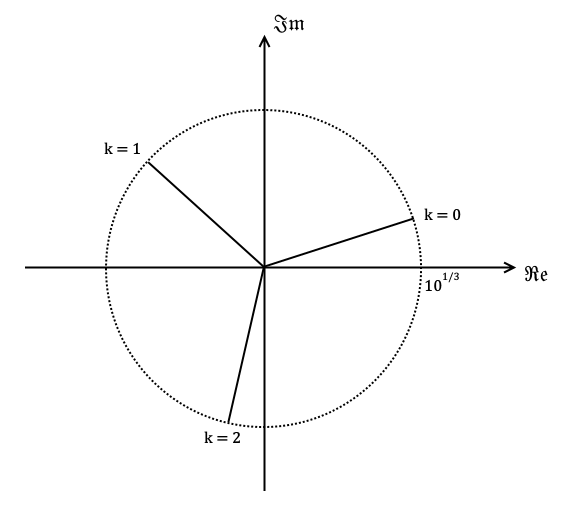### Roots of unity

A specialisation of finding $n^{th}$ roots of complex numbers is finding the $n^{th}$ roots of unity, where 'unity' is 1.

Let $$z^{n} = 1 = e^{2ik\pi}$$ where $k=0, 1, 2, \ldots$. Then taking the $n^{th}$ root of both sides

$$z = e^{i\frac{2k\pi}{n}}$$

Then to find all $n$ of the roots we sub in integer values of $k=0, 1, 2, \ldots$ and stop when we have all $n$ distinct roots.

### Example

Q) Find the fourth roots of unity.

A) Let

$$z^{4} = 1 = e^{2ik\pi}$$

Where $k=0, 1, 2, 3$. Then take the fourth roots of both sides

$$z = e^{i\frac{2k\pi}{4}} = e^{i\frac{k\pi}{2}}$$

$k=0$:

$$z = e^{i\frac{0\pi}{2}} = 1$$

$k=1$:

$$z = e^{i\frac{1\pi}{2}} = e^{i\frac{\pi}{2}}$$

$k=2$:

$$z = e^{i\frac{2\pi}{2}} = e^{i\pi} = -1$$

$k=3$:

$$z = e^{i\frac{3\pi}{2}}$$

All four of the roots have the same modulus, so they lie on a circle of radius 1 and centered at the origin going around the Argand diagram.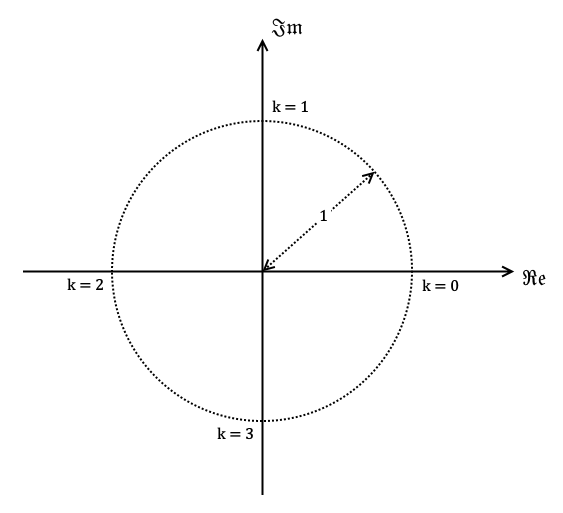## Loci on the complex plane

It's possible to express different shapes and transformations on the complex plane.

### Circle

This expresses all the points equidistant from some point $z_1$ on the complex plane. It can be thought of as a circle of radius $r$ and centered at $z_1$.

$$|z-z_1|=r$$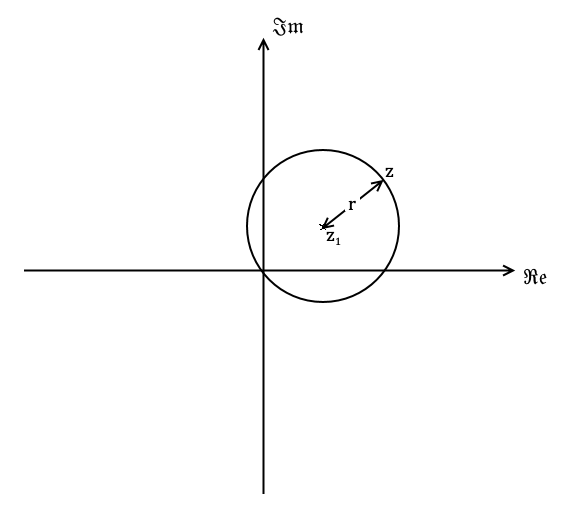Alternatively these expresses a 'shaded in' disc of points of radius $r$ centered at some point $z_1$ on the complex plane.

$$|z-z_1| \lt r \textrm{ and } |z-z_1| \le r$$The inclusive equality version also includes the set of points at the edge of the circle.

### Example

Q) Sketch $z$ such that $1\lt |z+3+4i| \lt 4$.

A) This is a 'frisbee' shape. Think of a disc radius 4 centered at $-3-4i$ with a disc of radius 1 centered at the same point cut out.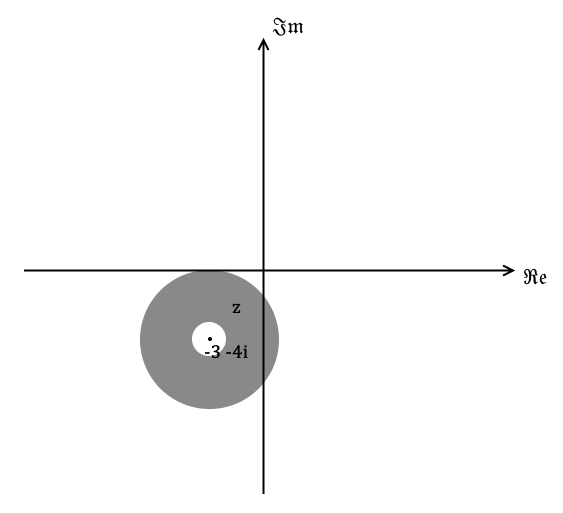### Perpendicular bisector

This expresses a straight line of all the points equidistant from two points $z_1$ and $z_2$ on the complex plane.

$$|z-z_1| = |z-z_2|$$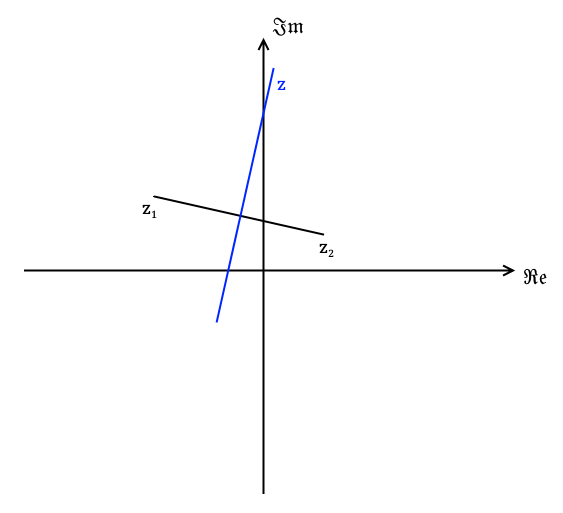### Example

Q) Find the locus of $z$ such that $|z-1| = |z-2+i|$.

A) Let $z=x+yi$

\begin{align} |x+yi-1| &= |x+yi-(2-i)| \\ |(x-1)+yi| &= |(x-2)+i(y+1)| \\ \Rightarrow \sqrt{(x-1)^{2}+y^{2}} &= \sqrt{(x-2)^{2}+(y+1)^{2}} \\ \Rightarrow (x-1)^{2}+y^{2} &= (x-2)^{2}+(y+1)^{2} \\ x^{2}-2x+1+y^{2} &= x^{2}-4x+4+y^{2}+2y+1 \\ -2x &= -4x + 4 + 2y \\ \therefore y &= x -2 \end{align}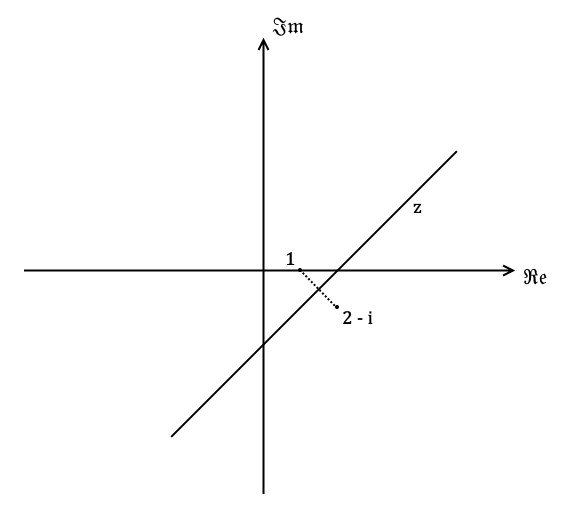### Half lines

This expresses a 'half line' of points with argument $\theta$. This can be quite hard to visualise. Think of a straight line of points going from $z$ on the line to a point $z_1$, such that every $z$ on the line has the specified argument $\theta$.

$$\arg(z-z_1) = \theta$$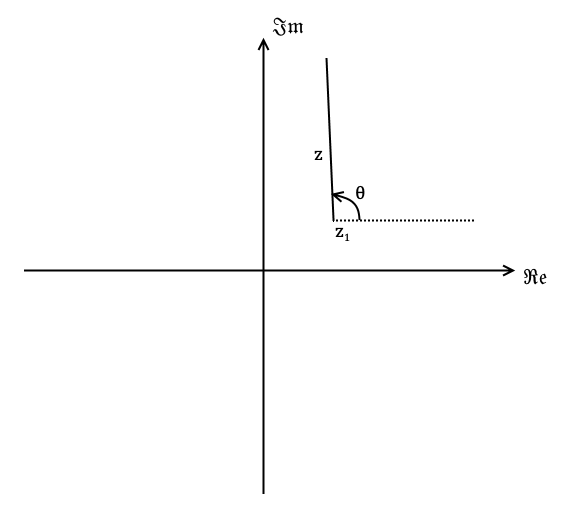### Example

Q) Find the locus of $z$ such that $\arg(z-1-i) = \frac{\pi}{6}$.

A) From the information given $z_1 = 1+i$ and we need $\arg(z-(1+i)) = \frac{\pi}{6}$.

Firstly $\tan\left(\frac{\pi}{6}\right) = \frac{1}{\sqrt{3}}$. Now let $z=x+yi$

$$\arg(x+yi-(1+i)) = \arg((x-1)+i\left(y-1\right))$$

Therefore

\begin{align} \frac{y-1}{x-1} &= \frac{1}{\sqrt{3}} \\ y - 1 &= (x-1)\frac{1}{\sqrt{3}} \\ \Rightarrow y &= 1 - \frac{1}{\sqrt{3}} + \frac{1}{\sqrt{3}}x \end{align}

And this is a half line so it starts from $1+i$ to ensure that the argument stays as $\frac{\pi}{6}$.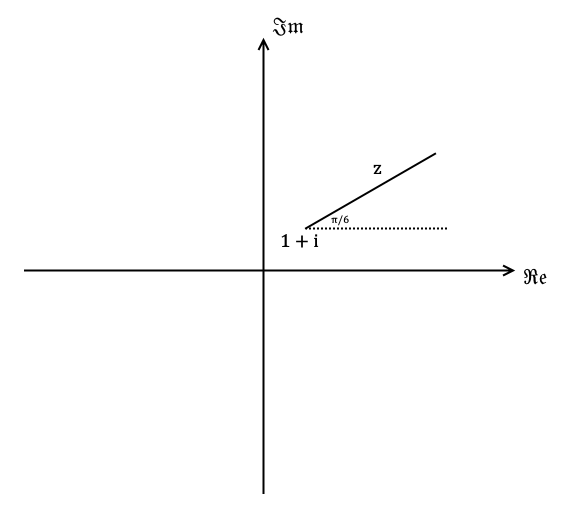### Example

Q) Find the locus of $z$ such that $\frac{\pi}{6} \le \arg(z-i) \le \frac{\pi}{3}$.

Firstly $\tan\left(\frac{\pi}{6}\right) = \frac{1}{\sqrt{3}}$ and $\tan\left(\frac{\pi}{3}\right) = \sqrt{3}$. Now let $z=x+yi$

$$\arg(x+yi-i) = \arg(x+i(y-1))$$

For the lower bound of the inequality

\begin{align} \frac{y-1}{x} &= \frac{1}{\sqrt{3}} \\ y - 1 &= \frac{1}{\sqrt{3}}x \\ \Rightarrow y &= 1 + \frac{1}{\sqrt{3}}x \end{align}

For the upper bound of the inequality

\begin{align} \frac{y-1}{x} &= \sqrt{3} \\ y - 1 &= \sqrt{3}x \\ \Rightarrow y &= 1 + \sqrt{3}x \end{align}

So the 'shaded in' argument area is bounded below by the line $y = 1 + \frac{1}{\sqrt{3}}x$ and bounded above by $y = 1 + \sqrt{3}x$.